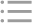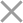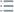## Fill out the form in 2 simple steps below:

Step 1

Step 2
** By clicking this button you agree to our Privacy Policy statementRequest our prices
--Select currency--
USD
EUR
* By clicking this button you agree to our Privacy Policy statementFree PVS‑Studio license for Microsoft MVP specialists
* By clicking this button you agree to our Privacy Policy statementTo get the licence for your open-source project, please fill out this form
* By clicking this button you agree to our Privacy Policy statementI am interested to try it on the platforms:
 Windows Linux macOS PVS-Studio for .NET Core JetBrains Rider
* By clicking this button you agree to our Privacy Policy statementMessage submitted.

Your message has been sent. We will email you at

check your Spam/Junk folder and click the "Not Spam" button for our message.
This way, you won't miss messages from our team in the future.

>
>
>
V550. Suspicious precise comparison. Co…Introduction
Analyzing projects
On Windows
On Linux and macOS
Cross-platform
IDE
Build systems
Game Engines
Continuous use of the analyzer in software development
Deploying the analyzer in cloud Continuous Integration services
Managing analysis results
Analyzer diagnostics
General Analysis (C++)
General Analysis (C#)
General Analysis (Java)
Micro-Optimizations (C++)
Micro-Optimizations (C#)
Diagnosis of 64-bit errors (Viva64, C++)
Customer specific requests (C++)
MISRA errors
AUTOSAR errors
OWASP errors (C++)
OWASP errors (C#)
OWASP errors (Java)
Problems related to code analyzerContents

# V550. Suspicious precise comparison. Consider using a comparison with defined precision: fabs(A - B) < Epsilon or fabs(A - B) > Epsilon.

Feb 26 2012

The analyzer detected a potential error: the == or != operator is used to compare floating point numbers. Precise comparison might often cause an error.

Consider this sample:

``````double a = 0.5;
if (a == 0.5)               //OK
x++;

double b = sin(M_PI / 6.0);
if (b == 0.5)               //ERROR
x++;``````

The first comparison 'a == 0.5' is true. The second comparison 'b == 0.5' may be both true and false. The result of the 'b == 0.5' expression depends upon the processor, compiler's version and settings being used. For instance, the 'b' variable's value was 0.49999999999999994 when we used the Visual C++ 2010 compiler. A more correct version of this code looks this way:

``````double b = sin(M_PI / 6.0);
if (fabs(b - 0.5) < DBL_EPSILON)
x++;``````

In this case, the comparison with error presented by DBL_EPSILON is true because the result of the sin() function lies within the range [-1, 1]. But if we handle values larger than several units, errors like FLT_EPSILON and DBL_EPSILON will be too small. And vice versa, if we handle values like 0.00001, these errors will be too big. Each time you must choose errors adequate to the range of possible values.

Question: how do I compare two double-variables then?

``````double a = ...;
double b = ...;
if (a == b) // how?
{
}``````

There is no single right answer. In most cases, you may compare two variables of the double type by writing the following code:

``````if (fabs(a-b) <= DBL_EPSILON * fmax(fabs(a), fabs(b)))
{
}``````

But be careful with this formula - it works only for numbers with the same sign. Besides, if you have a row with many calculations, there is an error constantly accumulating, which might cause the 'DBL_EPSILON' constant to appear a too small value.

Well, can I perform precise comparison of floating point values?

Sometimes, yes. But rather rarely. You may perform such comparison if the values you are comparing are one and the same value in its sense.

Here is a sample where you may use precise comparison:

``````// -1 - a flag that the variable's value is not set
float val = -1.0f;
if (Foo1())
val = 123.0f;
if (val == -1.0f) //OK
{
}``````

In this case, the comparison with value "-1" is permissible because it is this very value which we used to initialize the variable before.

We cannot cover the topic of comparing float/double types within the scope of documentation, so please refer to additional sources given at the end of this article.

The analyzer can only point to potentially dangerous code fragments where comparison may result unexpectedly. But it is only the programmer who may understand whether these code fragments really contain errors. We cannot also give precise recommendations in the documentation since tasks where floating point types are used are too diverse.

The diagnostic message isn't generated if two identical expressions of 'float' or 'double' types are being compared. Such a comparison allows to identify the value as NaN. The example of code implementing the verification of this kind:

``bool isnan(double X) { return X != X; }``

However, such code can't be called good. It's better to use the 'std::isnan' standard function instead.

References:

This diagnostic is classified as:

 CWE-682CERT-FLP02-C
 You can look at examples of errors detected by the V550 diagnostic.